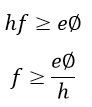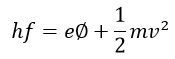# Photoelectric Emission

Definition: When the beam of the light enlightens the surface of the metals like sodium, potassium, caesium and rubidium then their electrons start emitting. The phenomenon of emission of electrons is known as the photoelectric emission.

In other words, the photoelectric emission is the process through which the free electrons are liberated from the surface of metals when it absorbs light. The photoelectric emission depends on the frequency of light and not on the intensity of light.

Some metals like alkaline, sodium, etc. require very less energy for moving the electrons from their surfaces. Or we can say their work function is very less. When the light energy incident on the surface of that metals, the electrons gets energised and starts emitting. The electrons which emitted through this way is known as the photoelectrons. And the current which induces because of the photoelectrons is known as the photoelectric current.

The number of electrons emitted depends on the intensity of light fall on the surface and the frequency of their radiations. This property of metals also used for measuring the intensity of light incident on the surface.

Consider the figure below shows the phenomenon of photoelectric emission. The emitter and the anode are enclosed in the evacuated glass tube G. The anode is made of thin metal and is kept at the positive potential concerning the emitter. When the light incident on the surface of metal then the electrons start ejected from the emitter surface. The electrons moved towards the positive anode and established the current in the external circuit. The flow of electrons is maintained till the illumination is continue.

###The energy of photons incident on the surface depends on the frequency of the light and is expressed as,where ‘h’ is the plank’s constant and their value is equal to the 6.626Χ10-34 joule-second, f shows the frequency of light in the hertz.

The energy requires for emission of electrons = eΦ

Where e – electrons charge and φ – work function of electrons in volts.

Hence the photons which cause emission is given by the expressionThe minimum amount of energy which causes the photoelectric emission is known as the threshold frequency. And it is expressed as,Where c is the velocity of light, and their value is equal to the 3 Χ 108 m/s.

The equations give the wavelength of the threshold frequency,If the radiation is higher then the above equation, the phenomenon of photoelectric emission will only occur when the magnitude of the wavelength is greater than the above equation. The energy relation becomes,Consider, the voltage V reduces the velocity of emitted electrons and in that case,The photoelectric emission phenomenon use in the phototubes and that tube is used in the TV and Films sounds.

### Laws of Photoelectric Emission

• The emission of electrons from the surface of the metal can only be done when the sufficient amount of energy incident on their surface.
• The number of electrons emitted per second is directly proportional to the amount of light incident on the surface.
• The energy of the emitted electrons is free from the amount of the photons incident on the surface, and it depends on the frequency of the light flux.

Note: If the electrons emitted from the surface of metal because of heating then the process is known as the thermionic emission.